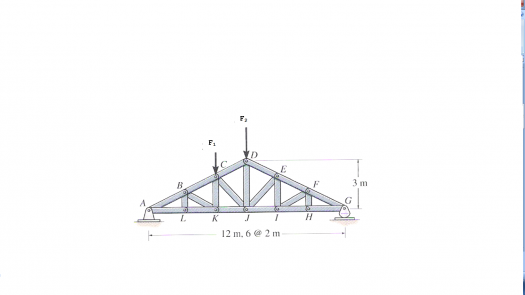# Strength of Materials (Stresses in a truss)

1 post / 0 new
Jal Rhee Ducusi...Strength of Materials (Stresses in a truss)

Mga idol. Pwede pa turo pano to? Thanks in advance.

Problem 1

For a truss loaded as show, choose the lightest 2-angle sections to safely carry the maximum stress. The yielding stress of steel is 240 MPa. Use allowable compressive stress of 0.45 of the yielding stress and allowable tensile stress of 0.60 of the yielding stress. Assume that provision for buckling is provided.
F1 = 15 KN; F2 = 14 KNFigure for Problem 1
Note: lightest section is the section with the least unit weight as indicated in the Table for Section Properties. (See portion for equal angles)

• Mathematics inside the configured delimiters is rendered by MathJax. The default math delimiters are $$...$$ and $...$ for displayed mathematics, and $...$ and $...$ for in-line mathematics.